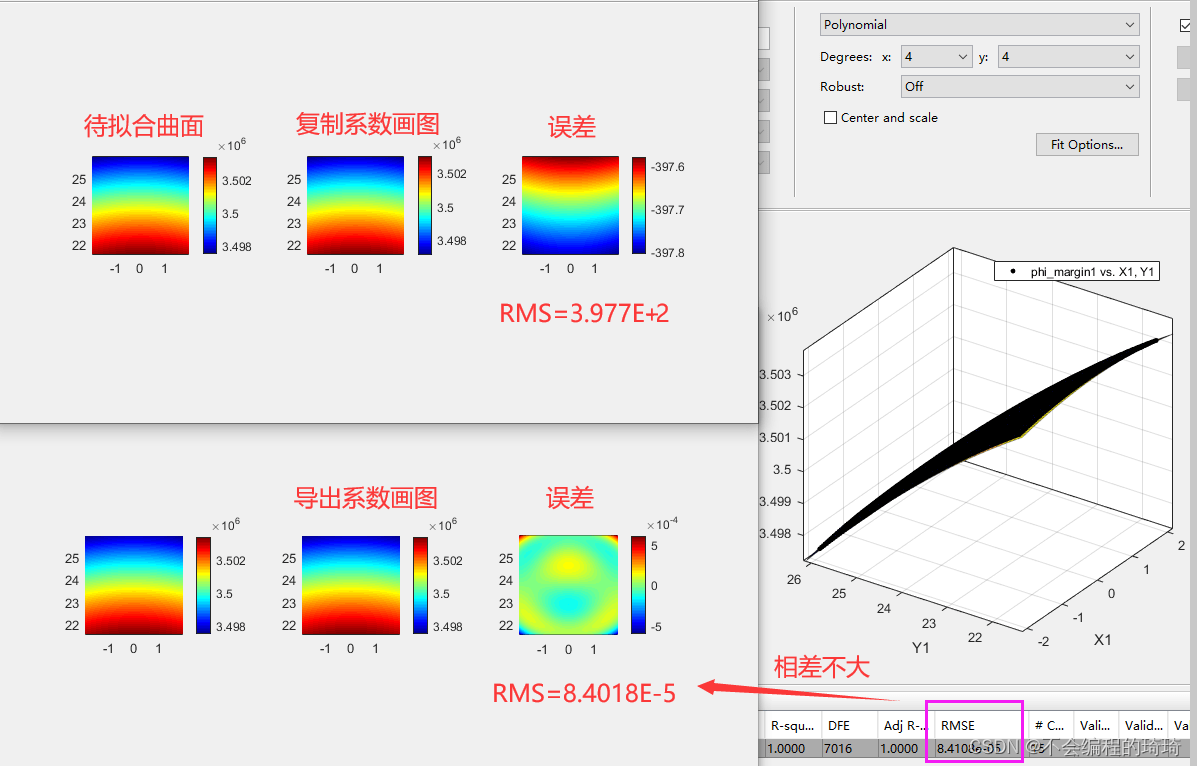# MATLAB-工具箱-如何导出拟合系数？由上图可知，使用导出系数画图与工具箱的RMES系数几乎一样。将会生成拟合函数，函数如：

``````function [fitresult, gof] = createFit(X, Y, phi_margin)
% Fit: 'untitled fit 1'.
[xData, yData, zData] = prepareSurfaceData( X， Y, phi_margin );

% Set up fittype and options.
ft = fittype( 'poly44' );%★这里我用的高次多项式拟合，x、y最高次数均为4次。

% Fit model to data.
[fitresult, gof] = fit( [xData, yData], zData, ft );

% Plot fit with data.
figure( 'Name', 'untitled fit 1' );
h = plot( fitresult, [xData, yData], zData );
legend( h, 'untitled fit 1', 'phi_margin vs. X, Y', 'Location', 'NorthEast' );
% Label axes
xlabel X
ylabel Y
zlabel phi_margin
grid on
view( -55.5, 30.8 );

``````

``````[fitresult, gof] = createFit(X, Y, phi_margin);%使用该函数
P= coeffvalues(fitresult);%输出系数``````